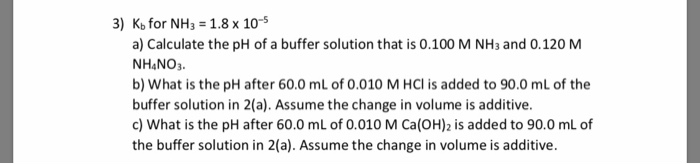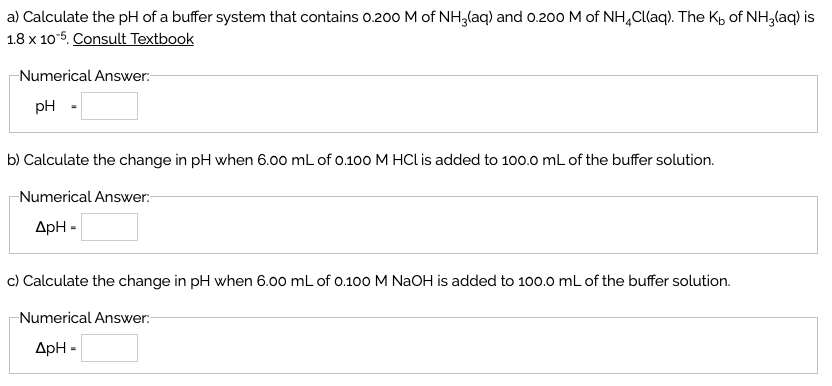Question

# A 0.500 L solution contains 1.68 g of NH3(aq) and 4.05 g of NH4CI(aq). Kb=1.80*10^-5 a.determine...

A 0.500 L solution contains 1.68 g of NH3(aq) and 4.05 g of NH4CI(aq). Kb=1.80*10^-5

a.determine the pH of this solution.

b.determine the volume of 6.0 M HCI(aq) that must be added to the above buffer solution to change the pH to9.00

a)pOH = pKb + log [BH+]/[B],

pH = 14.0 - pOH

[BH+] = moles of nh4cl / vol in litre

moles of nh4cl = 4.05/53.49 = 0.075

[BH+] = 0.151 M

[B] = (1.68/17)/0.500 = 0.197 M

pKb = - log[kb]

pOH = 4.74 + (-0.11) = 4.63

pH = 14-4.63 = 9.37

#### Earn Coins

Coins can be redeemed for fabulous gifts.

Similar Homework Help Questions
• ### 3) Kb for NH3 1.8 x 10-5 a) Calculate the pH of a buffer solution that...3) Kb for NH3 1.8 x 10-5 a) Calculate the pH of a buffer solution that is 0.100 M NH3 and 0.120 M NHANO3. b) What is the pH after 60.0 mL of 0.010 M HCl is added to 90.0 mL of the buffer solution in 2(a). Assume the change in volume is additive. c) What is the pH after 60.0 mL of 0.010 M Ca(OH)2 is added to 90.0 mL of the buffer solution in 2(a). Assume the change...

• ### a) Calculate the pH of a buffer system that contains o.200 M of NH3(aq) and o.200...a) Calculate the pH of a buffer system that contains o.200 M of NH3(aq) and o.200 M of NH4Cl(aq). The Kb of NH3(aq) is 18 x 10-5. Consult Textbook Numerical Answer pH b) Calculate the change in pH when 6.00 mL of 100 M HCl is added to 100.0 mL of the buffer solution. Numerical Answer: c) Calculate the change in pH when 6.00 mL of o.100 M NaOH is added to 100.0 mL of the buffer solution. Numerical Answer:

• ### How many grams of dry NH4Cl need to be added to 2.50 L of a 0.500 M solution of ammonia, NH3, to prepare a buffer soluti...

How many grams of dry NH4Cl need to be added to 2.50 L of a 0.500 M solution of ammonia, NH3, to prepare a buffer solution that has a pH of 8.74? Kb for ammonia is 1.8*10^-5.

• ### How many grams of dry NH4Cl need to be added to 1.50 L of a 0.500 M solution of ammonia, NH3,to prepare a buffer solutio...

How many grams of dry NH4Cl need to be added to 1.50 L of a 0.500 M solution of ammonia, NH3,to prepare a buffer solution that has a pH of 8.79? Kb for ammonia is 1.8*10^-5.

• ### How many grams of dry NH4Cl need to be added to 2.10 L of a 0.500 M solution of ammonia, NH3, to prepare a buffer solu...

How many grams of dry NH4Cl need to be added to 2.10 L of a 0.500 M solution of ammonia, NH3, to prepare a buffer solution that has a pH of 8.76? Kb for ammonia is 1.8×10−5. Express your answer with the appropriate units.

• ### Ammonia react with water as shown in the following equation: NH3(aq) + H2O(l)  NH4+(aq) +...

Ammonia react with water as shown in the following equation: NH3(aq) + H2O(l)  NH4+(aq) + OH-(aq) Kb = 1.77 x 10-5 and Ka = 5.65 x 10-10 a) Calculate the pH of the 0.030 M NH3 solution (Use a labeled ICE table) b) Calculate the pH of the solution at the equivalent point (when equal amount of acid is added. Assume the volume doubles). (Use a labeled ICE table) c) Over which pH range (actual numbers) do you expect...

• ### Calculate the pH of the following buffer system. 0.500 M NH3 ( Kb=1.8×10^(-5) ) 0.500 M...

Calculate the pH of the following buffer system. 0.500 M NH3 ( Kb=1.8×10^(-5) ) 0.500 M NH4 Br A. 4.74 B. 9.26 C. 7.00 D.0.30 E. 13.70 I got 4.74

• ### A 1.0-L buffer solution initially contains 0.25 mol of NH3 (Kb=1.76×10−5) and 0.25 mol of NH4Cl. In order to adjust the...

A 1.0-L buffer solution initially contains 0.25 mol of NH3 (Kb=1.76×10−5) and 0.25 mol of NH4Cl. In order to adjust the buffer pH to 8.85, should you add NaOH or HCl to the buffer mixture? What mass of the correct reagent should you add?

• ### a.Determine the pH of a 0.20 M CH3NH3Cl solution.    (Kb (CH3NH2) = 4.4 x 10-4)...

a.Determine the pH of a 0.20 M CH3NH3Cl solution.    (Kb (CH3NH2) = 4.4 x 10-4) b. Determine what products are expected to be produced (a) at the cathode and (b) at the anode as a result of electrolysis of an aqueous solution of MgI2 with inert electrodes. The standard electrode potentials are: O2(g) + 4 H+(aq) + 4 e-→ 2 H2O(l)                         E° = +1.23 V              I2(s) + 2 e- → 2 I-(aq)                                   E° = +0.54 V              2 H2O(l) + 2 e-...

• ### a) Calculate the pH of 0.500 L of a buffer solution that contains 0.200 M of...

a) Calculate the pH of 0.500 L of a buffer solution that contains 0.200 M of benzoic acid C6H5CO2H and 0.100 M sodium benzoate NaC6H5CO2. Ka = 6.3 x 10-5. b) Calculate the pH after .10 mL of 1.00 M H+ has been added. c) Calculate the pH after 10 mL of 1.00 M OH- has been added.